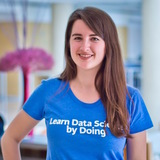Interactive Course

# Time Series Analysis in R

Learn the core techniques necessary to extract meaningful insights from time series data.

• 4 hours
• 16 Videos
• 58 Exercises
• 40,990 Participants
• 4,600 XP

### Loved by learners at thousands of top companies:### Course Description

Many phenomena in our day-to-day lives, such as the movement of stock prices, are measured in intervals over a period of time. Time series analysis methods are extremely useful for analyzing these special data types. In this course, you will be introduced to some core time series analysis concepts and techniques.

1. 1

#### Exploratory time series data analysis

Free

This chapter will give you insights on how to organize and visualize time series data in R. You will learn several simplifying assumptions that are widely used in time series analysis, and common characteristics of financial time series.

2. #### Correlation analysis and the autocorrelation function

In this chapter, you will review the correlation coefficient, use it to compare two time series, and also apply it to compare a time series with its past, as an autocorrelation. You will discover the autocorrelation function (ACF) and practice estimating and visualizing autocorrelations for time series data.

3. #### A simple moving average

In this chapter, you will learn the simple moving average (MA) model and several of its basic properties. You will also practice simulating and estimating the MA model in R, and compare the MA model with the autoregressive (AR) model.

4. #### Predicting the future

In this chapter, you will conduct some trend spotting, and learn the white noise (WN) model, the random walk (RW) model, and the definition of stationary processes.

5. #### Autoregression

In this chapter, you will learn the autoregressive (AR) model and several of its basic properties. You will also practice simulating and estimating the AR model in R, and compare the AR model with the random walk (RW) model.

1. 1

#### Exploratory time series data analysis

Free

This chapter will give you insights on how to organize and visualize time series data in R. You will learn several simplifying assumptions that are widely used in time series analysis, and common characteristics of financial time series.

2. #### Predicting the future

In this chapter, you will conduct some trend spotting, and learn the white noise (WN) model, the random walk (RW) model, and the definition of stationary processes.

3. #### Correlation analysis and the autocorrelation function

In this chapter, you will review the correlation coefficient, use it to compare two time series, and also apply it to compare a time series with its past, as an autocorrelation. You will discover the autocorrelation function (ACF) and practice estimating and visualizing autocorrelations for time series data.

4. #### Autoregression

In this chapter, you will learn the autoregressive (AR) model and several of its basic properties. You will also practice simulating and estimating the AR model in R, and compare the AR model with the random walk (RW) model.

5. #### A simple moving average

In this chapter, you will learn the simple moving average (MA) model and several of its basic properties. You will also practice simulating and estimating the MA model in R, and compare the MA model with the autoregressive (AR) model.

### What do other learners have to say?“I've used other sites, but DataCamp's been the one that I've stuck with.”

Devon Edwards Joseph

Lloyd's Banking Group“DataCamp is the top resource I recommend for learning data science.”

Louis Maiden“DataCamp is by far my favorite website to learn from.”

Ronald Bowers

Decision Science Analytics @ USAA##### David S. Matteson

Associate Professor at Cornell University

David S. Matteson is Professor of Statistical Science at Cornell University and co-author of Statistics and Data Analysis for Financial Engineering with R examples.

See More
##### Collaborators
•Lore Dirick

•Matt Isaacs